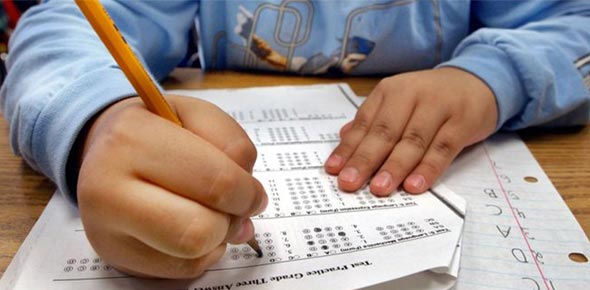# CRCT Grade 2 Math Review (3rd)

10 Questions | Total Attempts: 157Settings• 1.
64 people went to the park for a picnic.  The ice cream truck came with dessert,but only had 52 ice cream cones.  How many more ice cream cones are neededfor each person to have one for dessert?
• A.

116

• B.

12

• C.

16

• D.

20

• 2.
Look at the number sentence below.    5 + 4 = 7 + ___Which number makes the number sentence true?
• A.

9

• B.

16

• C.

2

• D.

0

• 3.
Mary and Joe watched the children on the playground.They wrote down how many people did each playground activity.They put the information in the chart below.GAMES PLAYED AT RECESS_______________________________________________Children who slid on the slide:            X  X  X  XChildren who jumped rope:                 X  X  XChildren who played on the swings:    X  X  X  X  X________________________________________________NOTE:  Each X stands for 5 students.How many students slid on the slide?
• A.

4

• B.

15

• C.

20

• D.

5

• 4.
• A.

2

• B.

1

• C.

5

• D.

4

• E.

12

• 5.
Which number is the same as sixty-three?
• A.

36

• B.

63

• C.

66

• D.

30

• 6.
What is the expanded form of 3,004
• A.

300 + 40

• B.

300 + 4

• C.

3,000 + 4

• D.

3,000 + 40

• 7.
Which number is even?
• A.

87

• B.

78

• C.

65

• D.

41

• 8.
Look at the number pattern below.7  *  10  *  13  *  A  *  B  *  22  *  25  *  C Where does the number 19 belong?
• A.

A

• B.

B

• C.

C

• 9.
What is the expanded form of 6,450
• A.

600 + 40 + 5

• B.

6,000 + 400 + 5

• C.

6,000 + 400 + 50

• D.

6,000 + 40 + 5

• 10.
Fred and Trudy went to the store to buy a book.Fred had 20 cents.  Trudy had 31 cents.The book they wanted cost 60 cents.Which number sentence tells the same math story?
• A.

20 + 31 + ___ = 60

• B.

60 + 20 = ___

• C.

20 + 31 + 60 = ___

• D.

20 + ___ = 31

Related TopicsBack to top